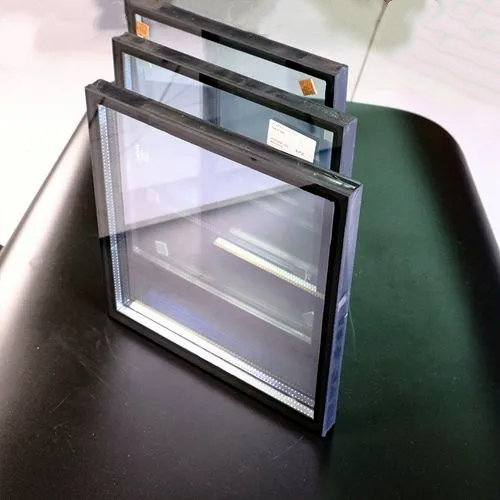#### 1. Preface

As the external maintenance structure of glass curtain wall buildings, glass doors and windows account for about 50% of the heat consumption of buildings, which has a great impact on the overall energy saving of glass buildings. And for the glass curtain wall commonly used in public buildings, the heat consumption of glass doors, windows, and glass curtain walls is higher than that of civil buildings, and the proportion of heat dissipation in building heat consumption will further increase. Therefore, as the material with the largest proportion of external protective area--glass, its energy-saving coefficient will become an important factor in the energy-saving of glass curtain wall buildings. In the high latitudes of the northern hemisphere, due to the relatively cold and long winter, the thermal insulation performance of glass is very important to the impact of building energy saving.

The thermal insulation performance of insulating glass is reflected by the heat transfer coefficient U value (standardized K value). In reality, the real-time U-value of insulating glass is a dynamic value that changes with the air and the environment. We cannot obtain all the real-time U-values of the environment where all the glass is located. The comparison of building energy-saving design requires a stable environment for comparison. The representative value of a specific environment is stipulated in the international industry standard "Calculation and Measurement of Insulating Glass Steady-state U Value (Heat Transfer Coefficient)". The U value of insulating glass is measured in this specific environment, and its level directly reflects whether Its thermal insulation performance is good or bad.

In addition, it should be noted that the U value of the insulating glass is the central U value of the insulating glass, which does not change with the size of the glass and the type of the spacer, and is a basic indicator of the thermal performance of the insulating glass. The standard stipulates that it refers to the heat passing through the unit area in the central area of the insulating glass (regardless of the edge effect), the two outer surfaces of the glass in the unit time and unit temperature difference under steady-state conditions, and the unit is W/ (m2・K).

Based on years of experience in the automatic production of insulating glass, LIJIANG Glass has determined that the U value of insulating glass can be obtained through theoretical calculation and physical measurement. Its measurement theory and software calculation are more suitable for early design guidance and are widely used. The international industry standard "Technical Regulations for the Application of Architectural Glass" lists the standard calculation method for the U value of insulating glass. LIJIANG Glass adopts this method for the calculation of the U value of double Low-E film insulating glass.

#### 2. The basic formula for heat transfer calculation of insulating glass

The heat transfer coefficient of insulating glass. The U value can be calculated according to the following formula: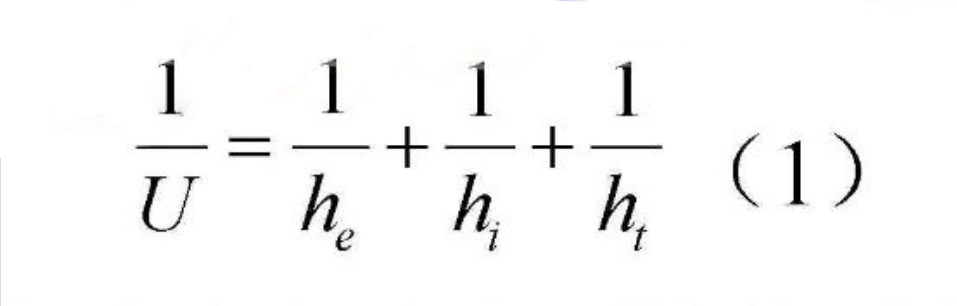In the formula:

he is the heat transfer coefficient of the glass outdoor surface ［W/ (m2・K)］;

his the surface heat transfer coefficient of the glass interior ［W/ (m2・K)］;

ht is the internal thermal conductivity of the multilayer glass system ［W/ (m2・K)］;

2.1 The calculation of indoor and outdoor heat transfer coefficient hi he

2.1.1 Outdoor surface heat transfer coefficient he

The outdoor surface heat transfer coefficient he is approximately calculated according to the industry standard and the formula he=10.0+4.1ν. In the formula, ν is the wind speed near the glass surface, in m/s; this calculation is based on 23 W/ (m2・K).

2.1.2 Indoor surface heat transfer coefficient hi

The indoor surface heat transfer coefficient hi is calculated approximately according to the industry standard, the formula hi=3.6+4.4ε/0.837. Where ε is the corrected emissivity of the glass interior surface. Uncoated ordinary transparent glass ε generally takes a value of 0.837, so hi can be taken as 8 W/ (m2・K). For the glass with a coating on the indoor surface, its corrected emissivity ε is multiplied by the standard emissivity εn according to the coefficient given by the industry standard

2.2 Internal heat transfer coefficient ht of multi-layer glass system

The internal heat transfer coefficient of the insulating glass system is calculated according to the following formula: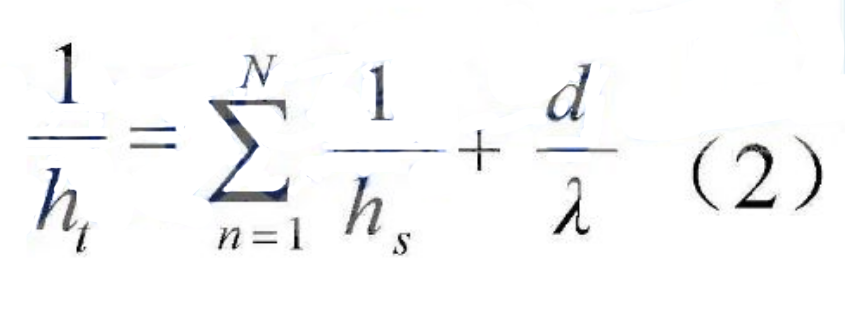In the formula:

ht is the thermal conductivity of the glass system [W/ (m2・K) ;

his the thermal conductivity of the insulating glass gas compartment ［W/(m2・K)］;

N is the number of gas layers of insulating glass;

λ is the glass thermal conductivity [W/ (m · K)];

d is the sum (m) of the thickness of every single piece of glass that makes up the glass system.

2.2.1 hs for thermal conductivity of insulating glass gas gap layer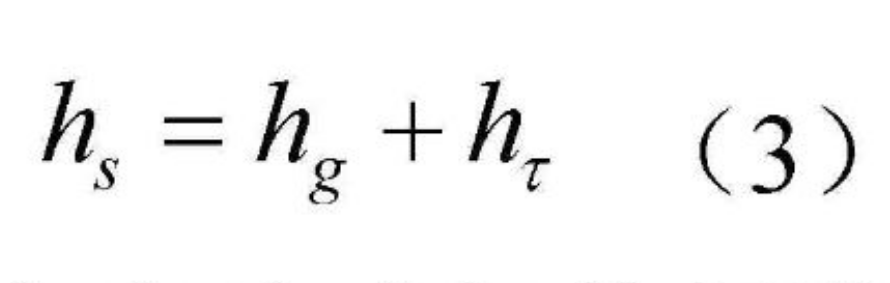In the formula:

hg is the gas thermal conductivity of the gas gap layer of the insulating glass [W/ (m · K)];

his the radiation heat conductance between two pieces of glass in the gas gap layer of

insulating glass [W/ (m · K)].

2.2.1.1 Gas thermal conductivity hg of insulating glass gas gap layer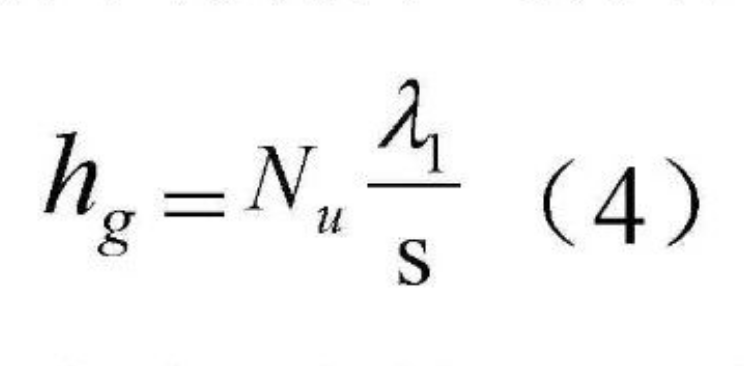In the formula:

Nμ is the Nusselt standard number;s is the thickness (m) of the gas spacer layer;

λis the gas thermal conductivity [W/ (m · K) ].

Nusselt's Quasi-Number: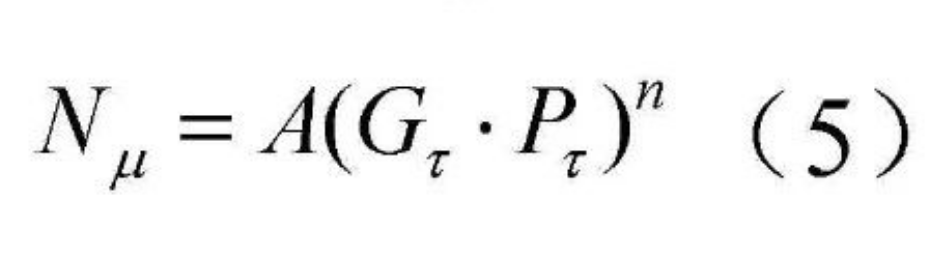In the formula:

A is a constant;

Gis the Grashov quasi-number;

Pr is the Prandtl standard number;

n is the raising index;

Grashof Quasi-Number: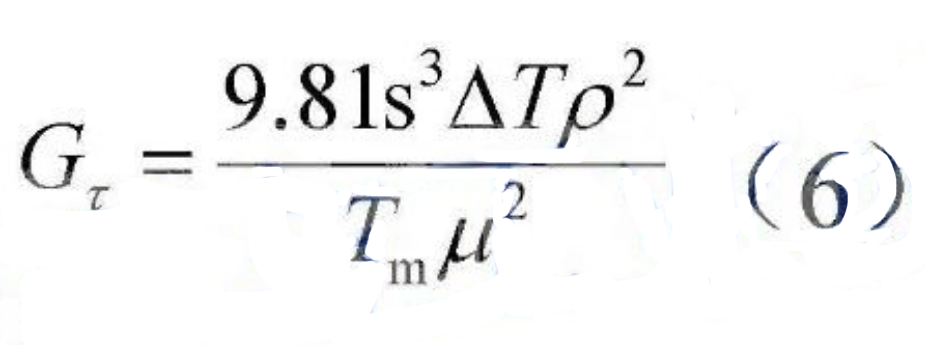Prandtl's number: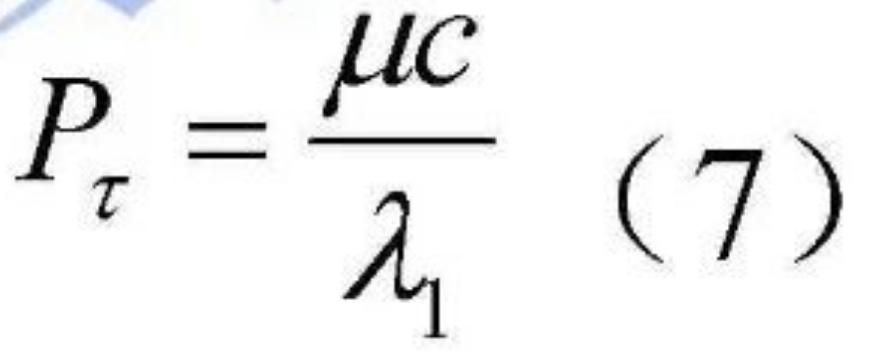In the formula:

ΔT is the temperature difference between the glass surfaces on both sides of the gas spacer;

ρ is the gas density;μ is the dynamic viscosity of gas;

c is the specific heat of the gas.

2.2.1.2 Radiation heat transfer coefficient hr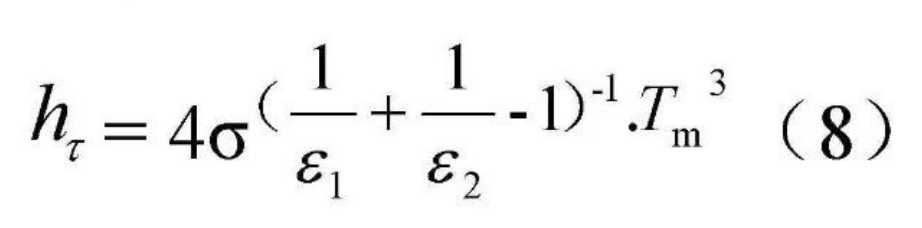In the formula:

σ is the Stefan-Boltzmann constant;

Tm is the average absolute temperature of the gas spacer;

ξ1, ξ2 are the corrected emissivity of the two glass surfaces in the spacer under the

average absolute temperature diagram;

3. Calculation and comparison of U value of double Low-E film single insulating glass

According to the current relevant national energy-saving policies, the impact of the change of the position of the second layer of Low-E film on the U value of the insulating glass is calculated with a representative glass configuration of 6 Low-E 1+12 air+6 Low-E 2 structure.
Design selection Low-E 1, standard emissivity εn=0.05, Low-E 2 standard emissivity εn=0.20.
Check relevant international industry standards;

ε1=0.05*1.18=0.059;

ε2=0.20*1.10=0.22.

3.1 Calculation structure 1: 6 Low-E 1#2+12 Air+6 Low-E 2#3

The value of σ is 5.67*10-8(w/m2・K);

Ttakes the average temperature of the insulating glass as 283 K; then it can be calculated:

h=0.250820345 (W/m*K);

P=0.711173077;

Gt=30.53920876;

N=0.112736518;

hg = 2.08 W/ (m2-K);

hs=2.330820345 W/ (m2-K)

The value of he is 23 W/ (m2-K);

Substitute the data into the formula: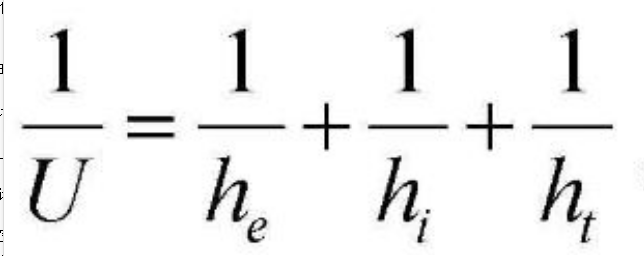Calculate the glass heat transfer coefficient U1=1.64 (w/m2・K).

3.2 Calculation structure 2:  6 Low-E1#2 + 12 Air + 6Low-E2#4

According to the method described above, the structure 2 glass heat transfer coefficient U=1.45 W/ (m2・K) can be calculated in the same way.

3.3 Calculation structure 3: 6 Low-E 1#2+12 Argon Gas+6 Low-E 2#3

When the gas in the insulated glass cavity is replaced with an inert gas, the characteristics of the gas in the insulating glass will change, and the gas density ρ, dynamic viscosity μ, thermal conductivity λ1, and specific heat capacity c need to be re-approved according to international industry standards.

The heat transfer coefficient U3=1.27 W/ (m2・K) of the glass of structure 3 is calculated in the same way.

3.4 Calculation structure 4: 6 Low-E 1#2+12 Argon Gas+6 Low-E 2#4

In the same way, the heat transfer coefficient of structure 3 glass U3=1.17 W/ (m2・K) is calculated.

3.5 comparison

List the calculation results as follows:

Table 1 Comparison of u value of double Low-E film and single insulating glass

 Argon-gas filling Heat transfer coefficient UW/ (m2・K) Structure 1Low-E 2#=3# surface Structure 2 Low-E2#=4# surface Structure 3 Low-E2#=3# surface Structure 4 Low-E2#=4# surface Air U1 1.64 - - - U2 - 1.45 - - Nitrogen U3 - - 1.27 - U4 - - - 1.17

When the insulating glass cavity is filled with air, the double low-emissivity film is arranged on the 2# and 3# surfaces of the insulating glass at the same time, and the heat transfer coefficient U value is 0.19 W/ (m2・K) , the Low-E film distributed on the 2#, 4# surface is 25% higher than the 2#, 3# surface insulation performance.

When the insulating glass cavity is filled with gas, the double low-emissivity film is arranged on the 2# and 3# inner surfaces of the insulating glass at the same time, and the heat transfer coefficient U value is 0.1 W/ (m2・K) higher than that distributed in the cavity 2# and indoor surface 4#, the Low-E film distributed on 2# and 4# surface is 7.8% higher than that of 2# and 3# surface.

#### 4. The conclusion

Through calculation, LIJIANG Glass concluded that for single-cavity insulating glass, the use of double Low-E film insulating glass can improve the thermal insulation performance of the glass. The second layer of low-emissivity film is placed on the side facing the room than in the cavity of the insulating glass, which can improve the overall energy-saving performance of the glass.

However, it should be noted that the common low-emissivity film layer containing a metallic silver layer is prone to chemical corrosion reaction if exposed to a humid air environment, and the film layer is prone to oxidation, peeling off, and loss of low-emissivity function. The low-emissivity film used in indoor exposure environments should be a functional film layer that is chemically stable, will not oxidize, and is easy to clean and maintain after use.